# Edge of Grid

When computing the Grids | Edit | Filter values of the output nodes near the edge of the grid, the specified neighborhood may extend outside the range of the grid. This presents an issue because the output grid nodes are then functions of non-existent input grid nodes. There are three general ways of dealing with this issue: assign the NoData value to the output grid nodes near the edge, modify the defining function near the edge, or generate artificial input grid nodes beyond the edge upon which the defining function operates. The following methods for handling the edge are as follows:

## Assign NoData

Any grid node, for which its neighborhood overlaps one or more edge, is assigned the NoData value. For example, if the neighborhood size is 3×3 then every application of the filter assigns the NoData value to one line of nodes on each edge. Every application of the filter shrinks the active grid by two rows and two columns: one row on the top, one row on the bottom, one column on the left edge, and one column on the right edge. Similarly, a 5×5 filter neighborhood Assigns the NoData value to four rows and four columns every application.

## Ignore

Ignore is the default setting. In this case, the filter function is modified by truncating the neighborhood at the edge of the grid. For example, in the Neighborhood section, even though the general neighborhood is specified to be 3×3, the effective neighborhood of node (21, 0), on the left edge of the grid, is only 3×2:

(22, 0) (22, 1)

(21, 0) (21, 1)

(20, 0) (20, 1)

This approach to handling the edges applies an essentially different function near the edges than in the middle of the grid. This can cause unexpected, visually apparent, artifacts in some extreme cases.

## Replicate

The edge grid node value is copied. For a grid with M rows and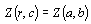where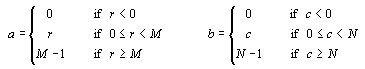## Mirror

The grid node value pattern at the edge is mirrored. For a grid with M rows andwhere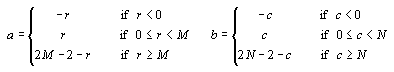## Cyclic Wrap

The grid is wrapped in two dimensions. If you go off the grid on the right edge, you come back on the left; if you go off on the top you come back on the bottom, etc. For a grid with M rows andwhere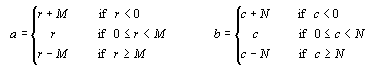## Fill

Fill the edge with a specified constant. A common value for the fill is the arithmetic average of the grid.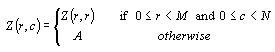where A is the user-specified constant. Enter a number into the Value box to the right when using this option.